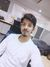# PROFET+2 open load in ON datasheet specification?Community ManagerCommunity Manager# PROFET+2 open load in ON datasheet specification?

The open load in ON state diagnostic sense current is specified in the datasheet at the point when the sense current is equal to 4uA. Given a typical sense resistor of 1.2Kohm, this would result in a diagnostic sense voltage of 4.8mV which is Infineon considers as the minimum value that a microcontroller ADC (Analog to Digital Converter) could measure in the application in order to use the current sensing diagnostic feature.

This condition is shown in the attachment P+2 Open Load in ON condition. The blue line would represent an ideal current sense characteristic, whereas the red lines represent the min/max behavior of all production devices.

How this is specified in the datasheet parameter table is shown in the attachment Open Load in ON-BTS7008-2EPA. This is an example from the BTS7008-2EPA datasheet. This parameter can be interpreted as the following: If the load current is less than 8mA it is guaranteed that the sense current will be less than 4uA, AND, if the load current is greater than 35mA it is guaranteed that the sense current will be greater than 4uA.

Thus effectively, in the low load current open load condition, the PROFET device ITSELF can distinguish a load current of 8mA from a load current of 35mA. However, in a real application there are additional error sources in the total system measurement for the sense current diagnostic feature, mainly the uC ADC +/- LSB (Least Significant Bit) error.

A detailed analysis of all errors in the total measurement system (uC ADC, resistor tolerances, Vreg tolerance, etc.) is required to determine the actual open load in ON state accuracy for the entire system.

129 Views